# Multiplication and Division of Whole Numbers

Students continue their work with multi-digit multiplication and division and the base-ten system in order to finalize fluency with multi-digit multiplication and extend division to two-digit divisors.

Math

Unit 2

## Unit Summary

In Unit 2, 5th grade students will build on their work on multi-digit multiplication and division from 4th grade as well as their understanding of the structure of the base-ten system in Unit 1 to develop fluency with multi-digit multiplication and extend multi-digit division to include two-digit divisors.

In 4th grade, students attained fluency with multi-digit addition and subtraction (4.NBT.4), a necessary skill for computing sums and differences in the standard algorithm for multiplication and division, respectively. Students also multiplied a whole number of up to four digits by a one-digit whole number, as well as two two-digit numbers (4.NBT.5). By the end of 4th grade, students can compute those products using algorithms, but “reason repeatedly about the connection between math drawings and written numerical work, help[ing] them come to see multiplication and division algorithms as abbreviations or summaries of their reasoning about quantities” (Progressions for the CCSSM, “Number and Operation in Base Ten, K-5", p. 14). Students also find whole-number quotients and remainders with up to four-digit dividends and one-digit divisors (4.NBT.6). Similar to multiplication, by the end of 4th grade, students can compute these quotients using algorithms alongside other strategies and representations so that the algorithms are meaningful rather than rote.

Unit 2 begins with writing, evaluating, and interpreting simple numerical expressions (5.OA.1, 5.OA.2). This serves both to review basic multiplication and division facts, which supports major content later on in the unit, and as a way to record calculations that will grow increasingly complex as the unit progresses. Then, students solidify the standard algorithm for multiplication with the computational cases from 4th grade before extending its use to larger and larger factors (5.NBT.5). Next, students follow a similar progression with division, first computing quotients involving cases from 4th grade using a variety of strategies and then extending those methods to computations involving two-digit divisors. Note, however, that unlike multiplication, fluency with the standard division algorithm is not expected until 6th grade (6.NS.2). Throughout the unit, students “learn to use [the] structure [of base-ten numbers] and the properties of operations to reduce computing a multi-digit…product or quotient to a collection of single-digit computations in different base-ten units” (MP.7) (Progressions for the CCSSM, “Number and Operation in Base Ten, K-5", p. 4). Further, “repeated reasoning (MP.8) that draws on the uniformity of the base-ten system is a part of this process” (Progressions for the CCSSM, “Number and Operation in Base Ten, K-5", p. 4).

Later this year, students will rely on their knowledge of whole-number computations to perform them with decimals (5.NBT.7). They will also write, evaluate, and interpret expressions with fractions and decimals in Unit 5 and Unit 6 (5.OA.1, 5.OA.2). This work "in Grade 5 prefigure[s] their later work with variable expressions" (6—8.EE) (OA Progression, p. 32). Further, students solidify fluency with the division algorithm and extend fluency with all four operations to decimals (6.EE.2—3). They also extend these computations to yet unknown parts of our number system, such as negative numbers (7.NS). Thus, by the end of this unit, students will be just shy of fluency with all four operations with whole numbers and ready to perform those operations with other types of numbers. They'll also be ready to think beyond calculation of numerical expressions to the understanding and interpretation of them, hinting at a new world of mathematics—algebra.

Pacing: 23 instructional days (20 lessons, 2 flex days, 1 assessment day)

Fishtank Plus for Math

Unlock features to optimize your prep time, plan engaging lessons, and monitor student progress.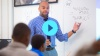## Assessment

The following assessments accompany Unit 2.

### Pre-Unit

Have students complete the Pre-Unit Assessment and Pre-Unit Student Self-Assessment before starting the unit. Use the Pre-Unit Assessment Analysis Guide to identify gaps in foundational understanding and map out a plan for learning acceleration throughout the unit.

### Mid-Unit

Have students complete the Mid-Unit Assessment after lesson 10.

### Post-Unit

Use the resources below to assess student understanding of the unit content and action plan for future units.

Expanded Assessment Package

Use student data to drive your planning with an expanded suite of unit assessments to help gauge students’ facility with foundational skills and concepts, as well as their progress with unit content.

## Unit Prep

### Intellectual Prep

Unit Launch

Prepare to teach this unit by immersing yourself in the standards, big ideas, and connections to prior and future content. Unit Launches include a series of short videos, targeted readings, and opportunities for action planning.#### Intellectual Prep for All Units

• Read and annotate the "Unit Summary" and "Essential Understandings" portions of the unit plan.
• Do all the Target Tasks in the unit and annotate them with the "Unit Summary" and "Essential Understandings" in mind.
• Take the Post-Unit Assessment.

#### Unit-Specific Intellectual Prep

• Students will use tape diagrams to represent expressions and contextual problems. They have used them extensively in previous grade levels to solve word problems. To learn more about using tape diagrams to model word problems, explore our Teacher Tool here: Modeling Word Problems with Tape Diagrams.
• Review the table below to familiarize yourself with the models used throughout the unit.
 area models Example: Solve 24 x 11 using an area model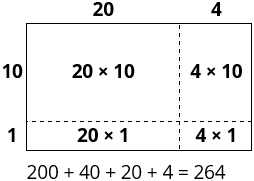partial products algorithm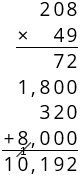standard algorithm for multiplication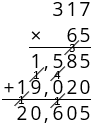partial quotients algorithm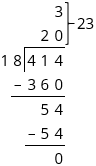standard algorithm for division/long division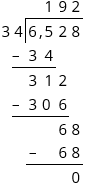### Essential Understandings

• When multiplying, it is more efficient to decompose the value with fewer non-zero digits. In the standard algorithm, this means writing the number with fewer non-zero digits on the bottom.
• When estimating with division, it is more effective to use numbers that are easy to compute mentally rather than rounded numbers (e.g., $${413 \div 64 \rightarrow 420 \div 60}$$).
• Some computational estimates can be better than others, depending on what numbers are chosen to use in place of the actual values. Computational estimates can be too high or too low, depending on how the numbers were originally estimated, the computation being performed, and where in the number sentence it’s located.
• General base-ten methods for multiplication and division (including the standard algorithm) are dependent on (1) an understanding how to compute products and quotients of multiples of 10, 100, 1,000, etc., and one-digit numbers, and (2) the distributive property.

### Vocabulary

equation

expression

grouping symbols (parentheses, brackets, braces)

To see all the vocabulary for Unit 2, view our 5th Grade Vocabulary Glossary.

## Unit Practice

Word Problems and Fluency Activities

Access daily word problem practice and our content-aligned fluency activities created to help students strengthen their application and fluency skills.## Lesson Map

Topic A: Writing and Interpreting Numerical Expressions

Topic B: Multi-Digit Whole Number Multiplication

Topic C: Multi-Digit Whole Number Division

## Common Core Standards

Key

Major Cluster

Supporting Cluster

### Core Standards

#### Number and Operations in Base Ten

• 5.NBT.B.5 — Fluently multiply multi-digit whole numbers using the standard algorithm.
• 5.NBT.B.6 — Find whole-number quotients of whole numbers with up to four-digit dividends and two-digit divisors, using strategies based on place value, the properties of operations, and/or the relationship between multiplication and division. Illustrate and explain the calculation by using equations, rectangular arrays, and/or area models.

#### Operations and Algebraic Thinking

• 5.OA.A.1 — Use parentheses, brackets, or braces in numerical expressions, and evaluate expressions with these symbols.
• 5.OA.A.2 — Write simple expressions that record calculations with numbers, and interpret numerical expressions without evaluating them. For example, express the calculation "add 8 and 7, then multiply by 2" as 2 × (8 + 7). Recognize that 3 × (18932 + 921) is three times as large as 18932 + 921, without having to calculate the indicated sum or product.

• 4.NBT.B.4
• 4.NBT.B.5
• 4.NBT.B.6
• 5.NBT.A.1
• 5.NBT.A.2

• 3.OA.D.8
• 4.OA.A.3

• 6.EE.A.2
• 6.EE.A.3
• 6.EE.A.4

• 6.NS.B.2
• 6.NS.B.3
• 6.NS.B.4

### Standards for Mathematical Practice

• CCSS.MATH.PRACTICE.MP1 — Make sense of problems and persevere in solving them.

• CCSS.MATH.PRACTICE.MP2 — Reason abstractly and quantitatively.

• CCSS.MATH.PRACTICE.MP3 — Construct viable arguments and critique the reasoning of others.

• CCSS.MATH.PRACTICE.MP4 — Model with mathematics.

• CCSS.MATH.PRACTICE.MP5 — Use appropriate tools strategically.

• CCSS.MATH.PRACTICE.MP6 — Attend to precision.

• CCSS.MATH.PRACTICE.MP7 — Look for and make use of structure.

• CCSS.MATH.PRACTICE.MP8 — Look for and express regularity in repeated reasoning.

Unit 1

Place Value with Decimals

Unit 3

Shapes and Volume

## Request a Demo

See all of the features of Fishtank in action and begin the conversation about adoption.

Yes

No

### Any other information you would like to provide about your school?